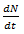## Chemistry Formulas for Nuclear Chemistry (Radioactivity)

Empirical relationship between size of nucleus and its mass number is
R = R0A1/3
Where,
A = mass number,
R0 = contestant = 1.4x10-13cm

### Rate of Decayof radioactive substanceWhere,
K = decay constant,
N = No. of atoms,
t = time of decay,
dN = small fraction of N,
dt = small fraction of t

### Value of Decay ConstantWhere,
N0 = No. of atoms originally present,
N = No. of atoms present after time t

### Half Life Time (t1/2)

t1/2 = 0.693/K
Where,
K = decay constant

### Average Life Time (T)

Average life time (T) =Sum of the lives of the nuclei/ Total number of nuclei
T = 1/K
Also,
Average life time (T) = 1.44 x Half-life (T1/2)Where,
K = decay constant
T = Average Life Time
T1/2 = Half Life

### Specific Activity

Specific Activity = Rate of decay/m
= KN/m
= K x Avogadro Number/ Atomic Mass in gram
Where,
N = Number of Radioactive nuclei that undergoes disintegration

Standard unit of radioactivity is curie (c).
1c = Activity of 1gram Ra226 = 3.7 x 1010dps
Where,
dps = disintegrations per second
millicurie (mc) = 3.7 x 107dps
microcurie (µc) = 3.7 x 104dps
Other units of radioactivity are Rutherford (rd) and Becquerel (Bq).

1rd = 106dps

#### Becquerel (Bq)

Becquerel (Bq) is the SI unit of radioactivity.
1Bq = 1 disintegrations per second
1 Bq = 1 dps